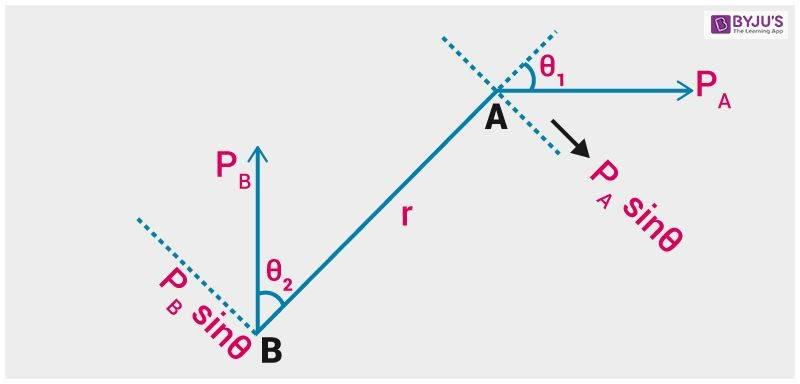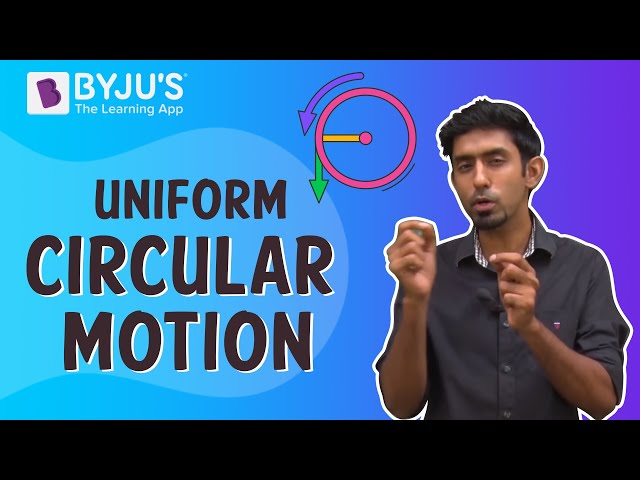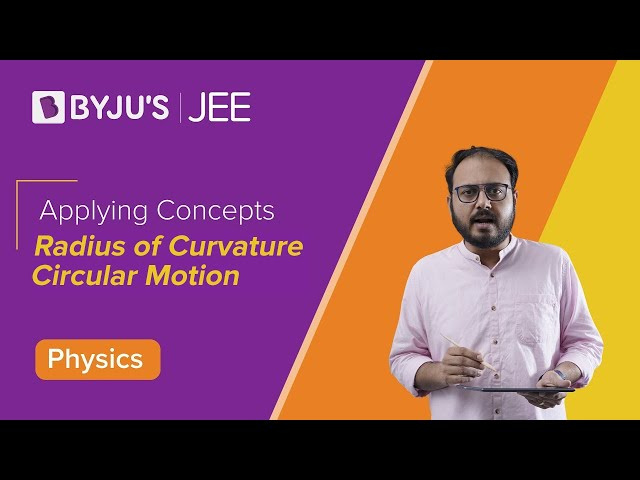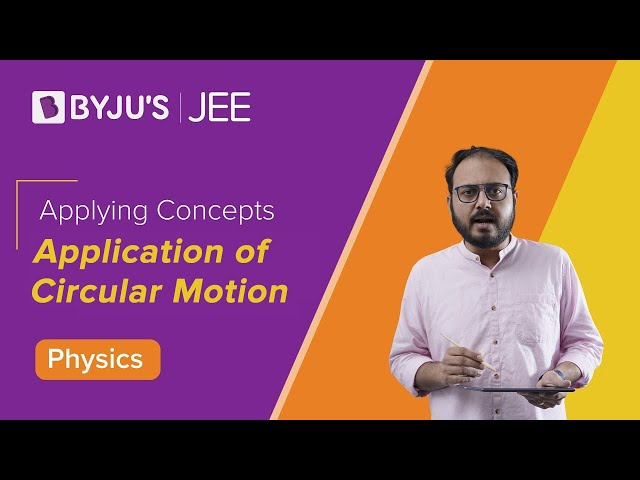Win up to 100% scholarship on Aakash BYJU'S JEE/NEET courses with ABNAT Win up to 100% scholarship on Aakash BYJU'S JEE/NEET courses with ABNAT

# Circular Motion IIT JEE Study Material

Circular motion is a motion in which the distance of a particle (radius) moving in a plane from a fixed point (centre) remains constant. The circular motion is a 2D motion in a plane. It can be classified into two types: uniform with constant speed and constant angular rotation rate and non-uniform with changing rotation rate.

## Variables of Motion

Angular Position: The angle subtended by the position vector with the reference line is called the angular position of a particle.

Angular Displacement (θ): It is the angle by which the position vector of the moving particle is rotated with respect to the reference line. It is the dimensionless quantity, and its SI unit is the radian. The angular displacement is also measured in degrees or revolutions.

$$\begin{array}{l}\mathbf{2\;\pi \;rad\;=\;360^{0}\;=\;1\;rev}\end{array}$$

Angular Velocity (ω): If θ1 and θ2 are angular positions of a particle at time t1 and t2, respectively, then the average angular velocity is given by:

$$\begin{array}{l}\omega_{av} = \frac{Total \; angle \; of \; rotation}{Total \; time \; taken} =\frac{\theta_2 – \theta_1}{t_{2} -t_{1}}=\frac{\Delta \theta }{\Delta t}\end{array}$$

A. Instantaneous Angular Velocity, i.e.

$$\begin{array}{l}\mathbf{\omega \;=\;\lim_{\Delta t \rightarrow 0}\;\frac{\Delta \; \theta }{\Delta \;t}\;=\;\frac{d \theta }{dt}}\end{array}$$

B. If θ = a – bt + ct2, then ω = dθ/dt = -b + 2 ct

C. If a body makes ‘n’ rotations in time t seconds, then the angular velocity:

$$\begin{array}{l}\mathbf{\omega _{av}\;=\;\frac{2\;\pi \;n}{t}}\ \text{radian sec}^{-1}\end{array}$$

D. It is an axial vector with dimensions T-1 and S.I. unit = rad sec-1.

Angular Acceleration (α): If ω1 and ω2 are angular velocities of a particle at time t1 and t2, respectively, then the average angular velocity is given by:

$$\begin{array}{l}\mathbf{\alpha _{av}\;=\;\frac{\omega _{2}\;-\;\omega _{1}}{t_{2}\;-\;t_{1}}\;=\;\frac{\Delta \omega}{\Delta t}}\end{array}$$

A. Instantaneous Angular Acceleration, i.e.

$$\begin{array}{l}\mathbf{\alpha \;=\;\lim_{\Delta t \rightarrow 0}\;\frac{\Delta \;\omega }{\Delta \;t}\;=\;\frac{d\;\omega }{dt}\;=\;\omega \;\;\frac{\Delta \;\omega}{\Delta \; \theta }}\end{array}$$

B. The motion is said to be the uniform circular motion if α = 0.

C.

$$\begin{array}{l}\mathbf{\omega \;=\;\frac{d \theta }{dt}\;\;and\;\;\alpha \;=\;\frac{d\;\omega }{dt}\;=\;\frac{d^{2}\; \theta }{dt^{2}}}\end{array}$$

D. It is an axial vector with dimensions as T-2 and the unit as rad sec-2.

## Uniform Circular Motion and Non-Uniform Circular Motion

If the particle moves in the circle with a uniform speed, we call it a uniform circular motion. In this case, the tangential component of acceleration is absent.

$$\begin{array}{l}\frac{dv}{dt} = 0\ \text{or}\ a_r = \omega^2 r\end{array}$$

In a non-uniform circular motion, the speed of the particle moving in a circle is not constant. In this case, the tangential component of acceleration is present.

$$\begin{array}{l}\frac{dv}{dt} = a_t\ \text{or}\ a_r = \omega^2 r\end{array}$$

In the case of non-uniform circular motion, the total acceleration is the result of the tangential and the radial acceleration.

## The Equations of Motion Associated with the Circular Motion

• $$\begin{array}{l}\mathbf{ \theta}=\mathbf{\omega_0 t+\frac{1}{2}at^2}\end{array}$$
• $$\begin{array}{l}\mathbf{\omega}=\mathbf{\omega_0+at}\end{array}$$
• $$\begin{array}{l}\mathbf{\omega^2}=\mathbf{\omega_0^2+2\alpha \theta}\end{array}$$

Where, ω0 and ω are the angular velocities at t = 0 and at time t, respectively, and θ is the angular position at any time.

## Velocity and Acceleration of a Particle in Circular Motion

Let at = tangential acceleration and ac = centripetal acceleration. Therefore, the net acceleration of the particle will be:

$$\begin{array}{l}\mathbf{a=\sqrt{a_{c}^{2}\;+\;a_{t}^{2}}=\sqrt{\left ( r\;\omega ^{2} \right )^{2}\;+\;\left ( \frac{dv}{dt} \right )^{2}}=\sqrt{\left ( \frac{v^{2}}{r} \right )^{2}\;+\;\left ( \frac{dv}{dt} \right )^{2}}}\end{array}$$

Where, v is the linear speed of the particle = r ω = relationship between speed and angular velocity.

And dv/dt is the rate of change of speed.

## Relative Angular Velocity

The relative angular velocity of an object P w.r.t. the other moving object Q is the angular velocity of the position vector of P w.r.t. Q.$$\begin{array}{l}\mathbf{\omega_{ab}\;=\;\frac{Relative\;\;velocity\;\;of\;\;P\;\;w.r.t.\;\;Q \;\;perp \;\;to \;\;line\;\;PQ}{Distance\;\;between\;\;P\;\;and\;\;Q}}\end{array}$$

i.e.,

$$\begin{array}{l}\mathbf{\omega _{PQ}\;=\;\frac{v_{P}\;Sin\; \theta _{1}\;+\;v_{Q}\;Sin\; \theta _{2}}{r}}\end{array}$$

## Dynamics of Circular Motion

In a circular motion, Newton’s law is applied in two perpendicular directions. The first one is along the tangent, and the other one is perpendicular to it (towards the centre). The component of force acting along the tangent is called the tangential force (Ft), and the component of force acting along the centre is called the centripetal force (Fc).

Tangential force

$$\begin{array}{l}\mathbf{F_{t}\;=\;M\;a_{t}\;=\;M\;\frac{dv}{dt}\;=\;M\;\alpha \;r}\end{array}$$

Centripetal force

$$\begin{array}{l}\mathbf{F_{c}\;=\;M\;a_{t}\;=\;m\;\omega ^{2}\;r\;=\;\frac{m\;v^{2}}{r}}\end{array}$$

Note: In the absence of the centripetal force, the object will move in a straight line with constant speed.

The radius of curvature of the instantaneous circle (R):

$$\begin{array}{l}\mathbf{R\;=\;\frac{\left [ 1\;+\;\left ( \frac{dy}{dx} \right )^{2} \right ]^{\frac{3}{2}}}{\left | \frac{d^{2}y}{dx^{2}} \right |}}\end{array}$$

## Motion in a Vertical Circle

Consider the motion of a stone tied to a string and whirled in a vertical circle. If at any time (t), the body is at the angular position θ, the forces acting are: tension (T) in the string along the radius (r) towards the centre and the weight (w) of the body, i.e., mg acting vertically downwards.

Now, applying Newton’s law towards the centre, we get,

$$\begin{array}{l}\mathbf{T\;-\;mg\;cos\; \theta \;=\;\frac{m\;v^{2}}{r}}\end{array}$$

Or,

$$\begin{array}{l}\mathbf{T\;=\;\;mg\;cos\; \theta \;+\;\frac{m\;v^{2}}{r}}\end{array}$$

Now, if Tmin > 0, the stone will move on the circular path.

And, if Tmin ≤ 0, the string will slack, and the body will fall down instead of moving on the circle.

Therefore, for looping the loop:

$$\begin{array}{l}\mathbf{\frac{m\;v_{H}^{2}}{r}\;-\;mg\;\geq \;0\;\;or\;\;v_{H}\;\geq \;\sqrt{gr}}\end{array}$$

Now, applying the conservation of mechanical energy between the lowest point (L) and the highest point (H):

$$\begin{array}{l}\mathbf{v_{L}\;\geq \;\sqrt{5\;gr}}\end{array}$$

Therefore, for looping the loop, the velocity at the lowest point must be greater than or equal to

$$\begin{array}{l}\mathbf{\sqrt{5\;gr}}.\end{array}$$

## Circular Motion Videos

### Uniform Circular Motion### Radius of Curvature – Circular Motion### Non-Uniform Circular Motion### Application of Circular MotionTest Your Knowledge On Circular Motion For Iit Jee!